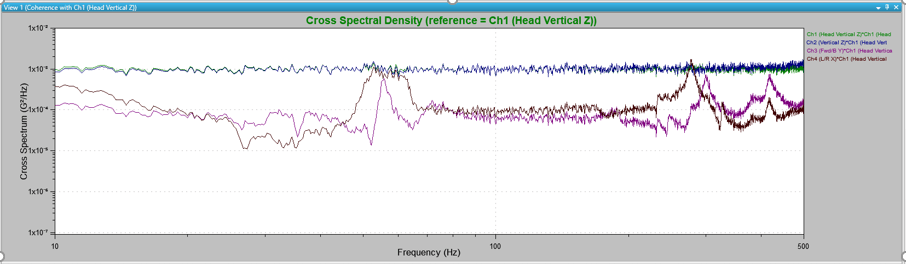# Cross Spectrum

January 3, 2019

The cross-spectrum density plot is a graph option available for random and shock test modules as part of the Analyzer package. The plot compares one signal with another signal in time. For example, the cross-spectral density may compare the drive signal with an input signal.

There may be a time delay in measurement when comparing signals from two different sources. Consequently, there also will be a phase difference between the two signals. Cross spectral density contains data regarding the differences in the amplitude of the two signals as well as the phase differences of the two signals.

The cross-spectrum plot is similar to the transfer function because they both provide information about amplitude and phase. However, a weighting function is applied during the cross-spectrum calculations. In practice, both are useful for gathering insight into testing behavior and should be used together.

### Importance of Cross Spectral Density

The cross-spectral density plot is primarily used to determine if there is a relationship between two variables. It is most beneficial when studying multi-axis systems. The cross-spectral density plot can determine how the vibrations in one axis are affecting the vibrations in another axis.

As an example, consider the following multi-axis system (Figure 2.6). An aluminum mass is mounted on a thin steel rod vibrating in the vertical axis.

A test engineer may ask, “Is the vibration in the x-axis influenced by the vibration in the z-axis?” As an object vibrates, it may be excited into a rotational or rocking motion. If this occurs, the object will have an x-axis component every time it is forced to vibrate in the z-axis. With a multi-axis vibration, the test engineer can use cross-spectral density to determine if there is a relationship between the motions.

In the following cross-spectral density plot, there is no significant signal in the x- or y-axes for a good portion of the spectrum. However, at approximately 60 Hz, 300 Hz, and 400 Hz, the vertical vibrations are exciting vibrations into the x- and y-axes (Figure 2.7).Figure 2.7. Cross-spectrum density plot for a multi-axis system. This plot demonstrates that the vibrations across the spectrum that were intended to affect the z-axis do affect the x- and y-axis at certain specific frequencies.

### Conclusion

The cross-spectrum density plot compares two signals, most commonly in multi-axis situations. A cross-spectrum density plot is commonly used to determine if the vibrations in one axis are affecting the vibrations in another axis.